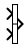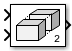Vector Concatenate, Matrix Concatenate

Concatenate input signals of same data type to create contiguous output signal

• Library:
• Simulink / Commonly Used Blocks

HDL Coder / Math Operations

HDL Coder / Signal Routing

••Description

The Vector Concatenate and Matrix Concatenate blocks concatenate the input signals to create a nonvirtual output signal whose elements reside in contiguous locations in memory.

In the Simulink® library, these blocks are different configurations of the same block. They differ based on the setting of their Mode parameter, which determines whether the block operates in vector or multidimensional array concatenation mode. The input signal requirements and output signal depend on the mode of concatenation.

BlockMode SettingInput Signal RequirementsOutput Signal
Vector ConcatenateVector

• Vectors

• Row vectors (1-by-M matrices)

• Column vectors (M-by-1 matrices)

• Combination of vectors and either row or column vectors

When all inputs are vectors, the output is a vector.

If any of the inputs are row or column vectors, the output is a row or column vector, respectively.

Tip

To create a vector of function calls, use a Mux block.

Matrix ConcatenateMultidimensional array

Signals of any dimensionality (scalars, vectors, and matrices)

The output is always an array.

Trailing dimensions are assumed to be 1 for lower dimensionality inputs. For example, if the output is 4-D and the input is [2x3] (2-D), this block treats the input as [2x3x1x1].

Concatenation is on the dimension that you specify with the Concatenate dimension parameter.

The signals in the output signal appear in the same order as the input signals for the block. For a description of the port order for various block orientations, see Port Location After Rotating or Flipping.

You can use multiple Vector Concatenate or Matrix Concatenate blocks to create the output signal in stages, but the result is flat, as if you used a single block to concatenate the signals.

You must use a Vector Concatenate or Matrix Concatenate block to define an array of buses. For more information, see Group Nonvirtual Buses in Arrays of Buses.

Ports

Input

expand all

First input to concatenate, specified as a scalar, vector, matrix, or N-D array.

• Inputs must be of the same data type.

• Matrix and N-D array inputs are supported only when you set Mode to Multidimensional array.

Data Types: single | double | half | int8 | int16 | int32 | int64 | uint8 | uint16 | uint32 | uint64 | Boolean | fixed point | enumerated | bus

Nth input to concatenate, specified as a scalar, vector, matrix, or N-D array.

• Inputs must be of the same data type.

• Matrix and N-D array inputs are supported only when you set Mode to Multidimensional array.

Dependencies

To enable this port, set Number of inputs to an integer greater than or equal to 2.

Data Types: single | double | half | int8 | int16 | int32 | int64 | uint8 | uint16 | uint32 | uint64 | Boolean | fixed point | enumerated | bus

Output

expand all

Concatenation of input signals along specified dimension. Outputs have the same data type as the input.

Data Types: single | double | half | int8 | int16 | int32 | int64 | uint8 | uint16 | uint32 | uint64 | Boolean | fixed point | enumerated | bus

Parameters

expand all

Specify the number of inputs for the block as a real-valued, positive integer, less than or equal to 65536.

Programmatic Use

 Block Parameter: NumInputs Type: character vector Values: positive integer Default: '2'

Select the type of concatenation that this block performs. The default Mode of the Vector Concatenate block is Vector. The default Mode of the Matrix Concatenate block is Multidimensional array.

• When you select Vector, the block performs vector concatenation.

• When you select Multidimensional array, the block performs matrix concatenation.

Mode SettingInput SignalsOutput
Vector

• Vectors

• Row vectors (1-by-M matrices)

• Column vectors (M-by-1 matrices)

• Combination of vectors and either row or column vectors

When all inputs are vectors, the output is a vector.

If any of the inputs are row or column vectors, the output is a row or column vector, respectively.

Multidimensional array

Signals of any dimensionality (scalars, vectors, and matrices)

The output is always an array.

Trailing dimensions are assumed to be 1 for lower dimensionality inputs. For example, if the output is 4-D and the input is [2x3] (2-D), this block treats the input as [2x3x1x1].

Concatenation is on the dimension that you specify with the Concatenate dimension parameter.

Programmatic Use

 Block Parameter: Mode Type: character vector Values: 'Vector' | 'Multidimensional array' Default: 'Vector'

Specify the output dimension along which to concatenate the input arrays.

• To concatenate input arrays vertically, enter 1.

• To concatenate input arrays horizontally, enter 2.

• To perform multidimensional concatenation on the inputs, specify an integer greater than 2.

If this parameter is set to 1 and the block inputs are 2-D matrices, the block performs vertical matrix concatenation and stacks the input matrices on top of each other to create the output matrix. For example, see the ex_concatenate_vertical model.If you set this parameter to 2 and the block inputs are 2-D matrices, the block performs horizontal matrix concatenation and places the input matrices side by side to create the output matrix. For example, see the ex_concatenate_horizontal model.The input matrices must have compatible sizes for concatenation. Vertical concatenation requires the input matrices to have the same number of columns. Horizontal concatenation requires input matrices to have the same number of rows.

If you set this parameter to 3 and the block inputs are 2-D matrices, the block performs multidimensional matrix concatenation. For example, see the ex_concatenate_multidims modelDependencies

To enable this parameter, set Mode to Multidimensional array.

Programmatic Use

 Block Parameter: ConcatenateDimension Type: character vector Values: scalar integer Default: '1'

Block Characteristics

 Data Types Boolean | double | enumerated | fixed point | half | integer | single Direct Feedthrough yes Multidimensional Signals no Variable-Size Signals yes Zero-Crossing Detection no